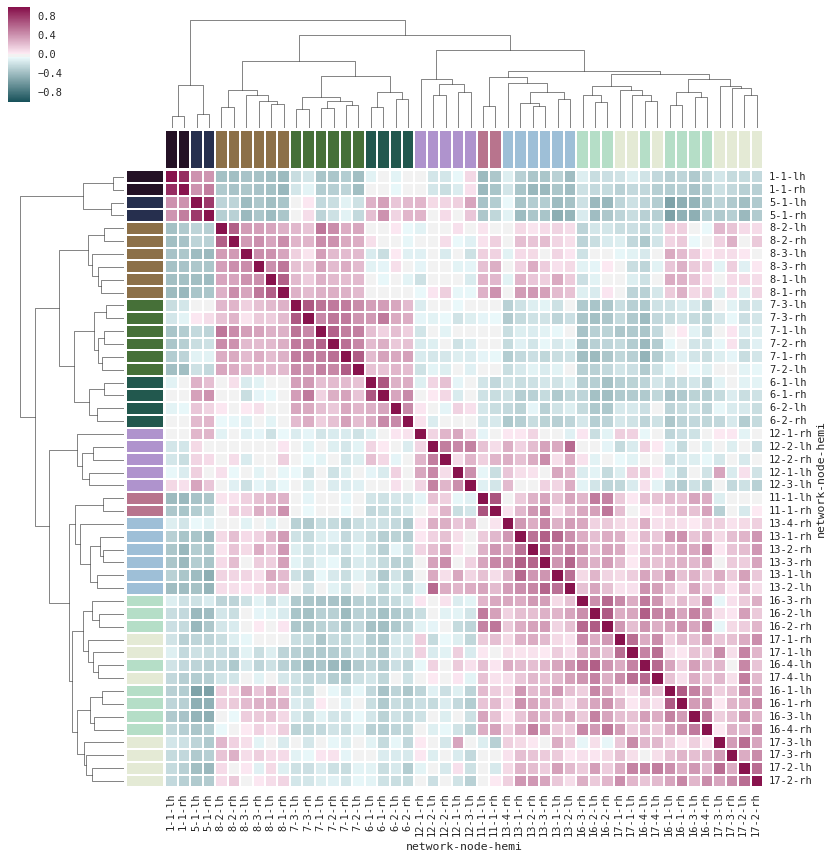# Stochastic Processes on Graphs 2015/16

Lecturer: Dr. Anton Klimovsky Contact
Time & Place: Wednesdays, 14-16 Uhr @ Raum WSC-N-U-4.04
Prerequisites: Probability Theory II
Target Audience: Master Math.
Load: 2 hrs/week (+ a Seminar)
Start: 21. Oktober### Stochastic Processes on Graphs

Over the past decade, networks became an extremely popular modeling paradigm. Here, behind each complex system, one often tries to find a network ($\approx$ graph) which encodes the interactions between the system's components.

In this course, we will get acquainted with the models of stochastic processes on graphs and methods to analyze them. We will deal with models, where the system's local variables live on the vertices of the graph. These local variables interact via the graph edges. While some of the ideas and terminology come from theoretical physics, such models are fundamental mathematical objects and are wide-spread in statistics, computer science, life sciences, etc.

We will learn about replica symmetry breaking, extremal processes, cavity method, local weak convergence, and (time permitting) message passing, Thouless-Anderson-Palmer (TAP) equations.

The course is followed by a concentrated seminar on the course topics (leading to 6 ECTS points).

### Topics:

• A short glimpse into Statistical Physics: Curie-Weiss model, Ising-like models on graphs, phase transitions, order parameters, mean-field equations, ground states, Gibbs measures, pure states, variational principles.
• Models with inhomogeneous interactions: range-free spin glasses, REM, GREM, Sherrington-Kirkpatrick model, Parisi theory, ultrametricity, Gibbs measures, variational principles.
• (Time permitting) Inference on/of networks: message passing, TAP-like equations.

### Literature

Research articles ($\approx$ seminar topics):